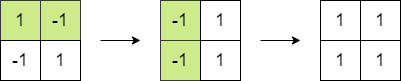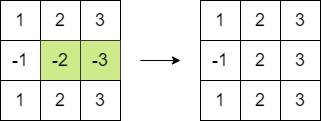# 最大方阵和

## 1975. 最大方阵和 (Medium)

• 选择 `matrix` 中 相邻 两个元素，并将它们都 乘以 `-1` 。```输入：matrix = [[1,-1],[-1,1]]

- 将第一行的 2 个元素乘以 -1 。
- 将第一列的 2 个元素乘以 -1 。
``````输入：matrix = [[1,2,3],[-1,-2,-3],[1,2,3]]

- 将第二行的最后 2 个元素乘以 -1 。
```

• `n == matrix.length == matrix[i].length`
• `2 <= n <= 250`
• `-105 <= matrix[i][j] <= 105`

[贪心] [数组] [矩阵]